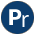#when.pl -- Conditional coroutining

This library implements the when/2 constraint, delaying a goal until its arguments are sufficiently instantiated. For example, the following delayes the execution of =:=/2 until the expression is instantiated.

```    ...
when(ground(Expr), 0 =:= Expr),```
author
- Tom Schrijvers (initial implementation)
- Jan Wielemaker
when(+Condition, :Goal)
Execute Goal when Condition is satisfied. I.e., Goal is executed as by call/1 if Condition is true when when/2 is called. Otherwise Goal is delayed until Condition becomes true. Condition is one of the following:
• `nonvar(X)`
• `ground(X)`
• ?=(X,Y)
• (Cond1,Cond2)
• (Cond2;Cond2)

For example (note the order `a` and `b` are written):

```?- when(nonvar(X), writeln(a)), writeln(b), X = x.
b
a
X = x```
\$eval_when_condition(+Condition, -Optimised)[private]
C-building block defined in pl-attvar.c. It pre-processes the when-condition, checks it for errors (instantiation errors, domain-errors and cyclic terms) and simplifies it. Notably, it removes already satisfied conditions from Condition, unifying Optimised to `true` if there is no need to suspend. Nested disjunctions are reported as `or(List)`.
trigger_ground(@Term, :Goal)[private]
Trigger Goal when Term becomes ground. The current implementation uses nonground/2, waiting for an atribtrary variable and re-check Term when this variable is bound. Previous version used term_variables and suspended on a term constructed from these variables. It is clear that either approach performs better on certain types of terms. The term_variables/2 based approach wins on large terms that are almost ground. Possibly we need a nonground that also returns the number of tests performed and switch to the term_variables/2 based approach if this becomes large.
check_disj(DisjVar, Disj, Goal)[private]
If there is a disjunctive condition, we share a variable between the disjunctions. If the goal is fired due to one of the conditions, the shared variable is boud to (-). Note that this implies that the attributed variable is left in place. The predicate when_goal//1 skips such goals on behalfe of copy_term/3.